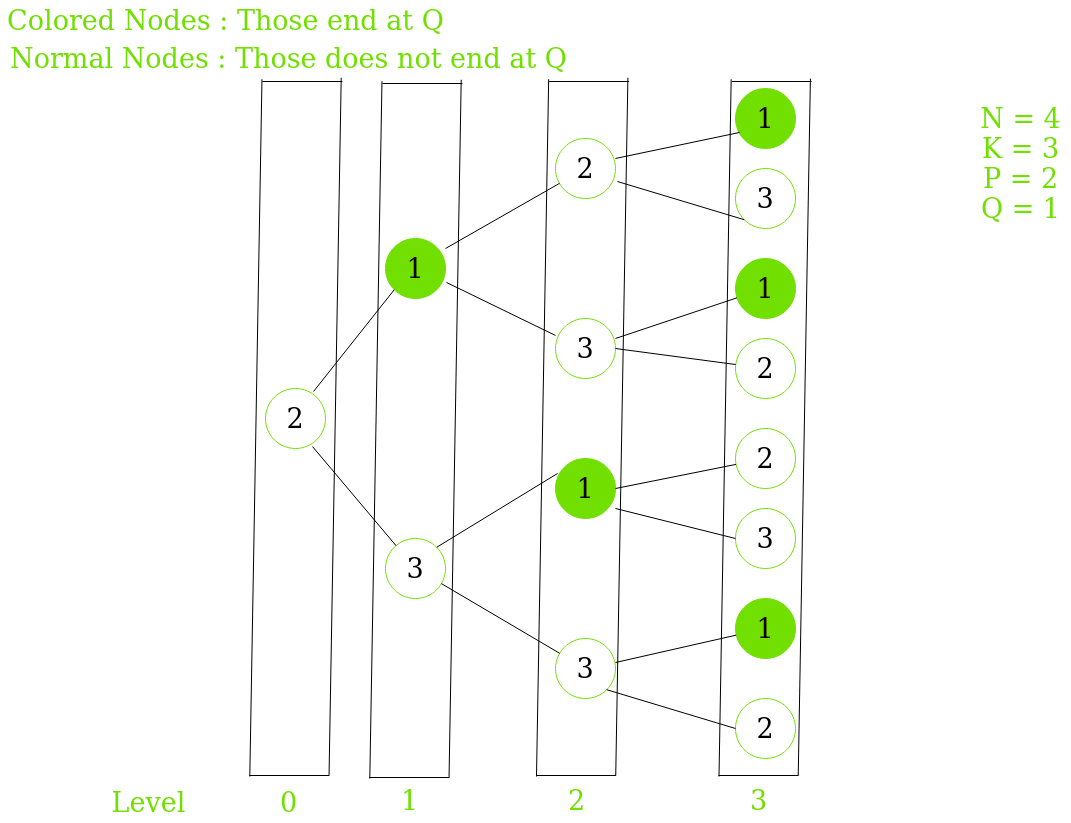# Number of ways to arrange N numbers which are in a range from 1 to K under given constraints.

Given Four integers N, K, P and Q. The task is to calculate the number of ways to arrange N numbers which are in a range from 1 to K such that the first number is P, the last number is Q and no two adjacent numbers are consecutive.
Examples:

```Input:  N = 4, K = 3, P = 2, Q = 3
Output: 3
Explanation:
For N=4, K=3, P=2, Q=3,
ways are [2, 1, 2, 3], [2, 3, 1, 3], [2, 3, 2, 3]

Input:  N = 5, K = 3, P = 2, Q = 1
Output: 5
```

Approach: The idea is to use Dynamic Programming to solve this problem.

• Let’s try to understand this by taking an example, N = 4, K = 3, P = 2, Q = 1.
We will observe all possible arrangements staring from P and try to find any pattern that can be useful to apply Dynamic programming.
• Below is the image showing all possible arrangements starting from P = 2.• Let A be the array that consists of the number of nodes ending at Q at a particular level
A = { 0, 1, 1, 3 }
Let B be the array that consists of the number of nodes NOT ending at Q at a particular level
B = {1, 1, 3, 5 }
• On carefull observation it may be noted that:
1. A[i] = B[i-1]
Reason :
All the favourable nodes ( ending at Q ) will only be produced by non-favourable nodes(NOT ending at Q) of the previous level.

2. B[i] = A[i-1]*(K – 1) + B[i-1]*(K – 2)
Reason :
• For A[i-1]*(K – 1), some of the non-favourable nodes are produced by favourable nodes of the previous level, multiply by (K – 1) as each favourable node will produce K-1 non-favourable nodes
• For B[i-1]*(K – 2), rest of the non-favourable nodes are produced by non-favourable nodes of the previous level, multiply by (K-2), as one produced node is favourable, so we subtract 2 from this.

## C++

 `// C++ program to calculate Number of  ` `// ways to arrange N numbers under ` `// given constraints. ` `#include ` `using` `namespace` `std; ` ` `  `class` `element { ` `public``: ` `    ``// For favourable nodes  ` `    ``// (ending at Q) ` `    ``int` `A; ` `     `  `    ``// For Non-favourable nodes ` `    ``// (NOT ending at Q) ` `    ``int` `B; ` `}; ` ` `  `// Function to print Total number ` `// of ways ` `void` `NumberOfWays(``int` `n, ``int` `k, ``int` `p,  ` `                                ``int` `q) ` `{ ` `    ``element* dp = ``new` `element[n]; ` ` `  `    ``// If the First number and the ` `    ``// last number is same. ` `    ``if` `(p == q) { ` `        ``dp.A = 1; ` `        ``dp.B = 0; ` `    ``} ` `    ``else` `    ``{ ` `        ``dp.A = 0; ` `        ``dp.B = 1; ` `    ``} ` ` `  `    ``// DP approach to find current state  ` `    ``// with the help of previous state. ` `    ``for` `(``int` `i = 1; i < n; i++) ` `    ``{ ` `        ``dp[i].A = dp[i - 1].B; ` `        ``dp[i].B = (dp[i - 1].A * (k - 1)) ` `                 ``+ (dp[i - 1].B * (k - 2)); ` `    ``} ` `     `  `    ``cout << dp[n - 1].A << endl; ` ` `  `    ``return``; ` `} ` ` `  `// Driver code ` `int` `main() ` `{ ` `    `  `   ``int` `N = 5; ` `   ``int` `K = 3; ` `   ``int` `P = 2; ` `   ``int` `Q = 1; ` `     `  `   ``// Function call ` `   ``NumberOfWays(N, K, P, Q); ` `} ` `    `

## Java

 `// Java program to calculate number of   ` `// ways to arrange N numbers under  ` `// given constraints.  ` `import` `java.io.*; ` `import` `java.util.*;  ` ` `  `class` `GFG{ ` ` `  `// Function to print Total number  ` `// of ways  ` `static` `void` `NumberOfWays(``int` `n, ``int` `k,  ` `                         ``int` `p, ``int` `q)  ` `{  ` `    ``int``[][] dp = ``new` `int``[n][``2``];  ` ` `  `    ``// If the First number and the  ` `    ``// last number is same.  ` `    ``if` `(p == q)  ` `    ``{  ` `        ``dp[``0``][``0``] = ``1``;  ` `        ``dp[``0``][``1``] = ``0``;  ` `    ``}  ` `    ``else` `    ``{  ` `        ``dp[``0``][``0``] = ``0``;  ` `        ``dp[``0``][``1``] = ``1``;  ` `    ``}  ` ` `  `    ``// DP approach to find current state  ` `    ``// with the help of previous state.  ` `    ``for``(``int` `i = ``1``; i < n; i++)  ` `    ``{  ` `        ``dp[i][``0``] = dp[i - ``1``][``1``];  ` `        ``dp[i][``1``] = (dp[i - ``1``][``0``] * (k - ``1``)) + ` `                   ``(dp[i - ``1``][``1``] * (k - ``2``));  ` `    ``}  ` `    ``System.out.println(dp[n - ``1``][``0``]);  ` `}  ` ` `  `// Driver Code  ` `public` `static` `void` `main(String args[])  ` `{  ` `    ``int` `N = ``5``;  ` `    ``int` `K = ``3``;  ` `    ``int` `P = ``2``;  ` `    ``int` `Q = ``1``;  ` `         `  `    ``// Function call  ` `    ``NumberOfWays(N, K, P, Q);  ` `} ` `}  ` ` `  `// This code is contributed by offbeat `

Output:

```5
```

Time Complexity: O(N).My Personal Notes arrow_drop_upCheck out this Author's contributed articles.

If you like GeeksforGeeks and would like to contribute, you can also write an article using contribute.geeksforgeeks.org or mail your article to contribute@geeksforgeeks.org. See your article appearing on the GeeksforGeeks main page and help other Geeks.

Please Improve this article if you find anything incorrect by clicking on the "Improve Article" button below.

Improved By : offbeat